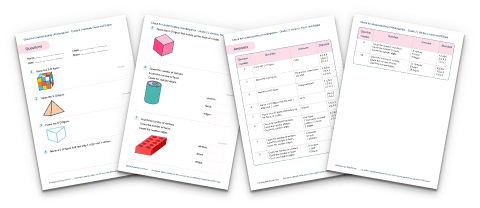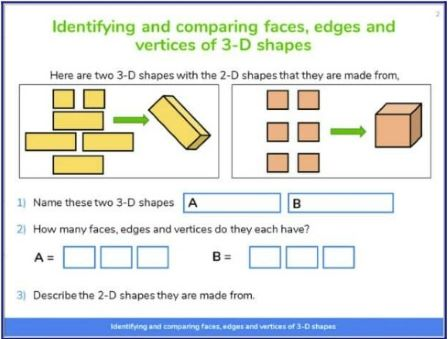Math teaching support you can trustone-on-one tutoring sessionsschools supported

[FREE] Themed Math Problems: Fall Term (Sep – Dec)

5th grade math problems and answers that link to key dates in the Fall Term, including Christmas!

# What Are Vertices, Faces And Edges? A Guide For Teachers

Vertices, faces and edges come up a lot in geometry when children are learning about the properties of 3d shapes. Here we explain what each of these mean and how to work out the number of vertices, faces and edges for any shape. We also include the number of edges, faces and vertices of the most common shapes.

### What are vertices in shapes?

Vertices in shapes are the points where two or more line segments or edges meet (like a corner). The singular of vertices is vertex. For example, a cube has 8 vertices and a cone has one vertex.

Vertices are sometimes called corners but when dealing with 2d and 3d shapes, the word vertices is preferred.

A cube has 8 vertices. 7 are visible here and one is hidden.Vertices, Faces and Edges Check for Understanding Quiz

Wondering if your students have fully grasped vertices, faces and edges? Use this quiz to check their understanding across 10 questions with answers.DOWNLOAD FREE

### What are edges?

Edges are the line segments that join one vertex to another and are also where the shape’s faces meet. These can be used to describe 2d and 3d shapes.

Although many shapes have straight lines and straight edges, there are shapes which have curved edges, such as a hemisphere and a cylinder. A cube will have 12 straight edges as seen below; 9 are visible and 3 are hidden.

### What are faces?

Faces are the flat surface of a solid shape. For example, a cuboid (rectangular prism) has 6 faces. When thinking about 2d and 3d shapes, it is important to know that a 2d shape merely represents the face of a 3d shape.

It is also important to know that as our reality is constructed in 3 dimensions, it is impossible to physically handle 2d shapes as we are surrounded by 3-dimensional shapes. Therefore, if in your classroom there is a drawer labeled ‘2d Shapes’ this should be removed as it is teaching children a misconception. Although an interactive concept for the classroom, 2d shapes can only exist as 2-dimensional drawings.

You can have both flat faces and curved faces, but I find it helpful to refer to curved faces as curved surfaces as it matches well with the visual of the shape.

There are three faces that are visible and three that are hidden on the cube below.

### Vertices, faces and edges of common 3d shapes

#### How many faces, edges and vertices does a cuboid (rectangular prism) have?

A cuboid (rectangular prism) has 8 vertices.

A cuboid (rectangular prism) has 12 edges.

A cuboid (rectangular prism) has 6 faces.

#### How many faces, edges and vertices does a cylinder have?

A cylinder has 0 vertice.

A cylinder has 2 edges.

A cylinder has 2 faces and 1 curved surface.

#### How many faces, edges and vertices does a hemisphere have?

A hemisphere has 0 vertice.

A hemisphere has 1 curved edge.

A hemisphere has 1 face and 1 curved surface.

#### How many faces, edges and vertices does a cone have?

A cone has 1 vertex.

A cone has 1 edge.

A cone has 1 face and 1 curved surface.

#### How many faces, edges and vertices does a tetrahedron (triangular pyramid)have?

A tetrahedron (triangular pyramid) has 4 vertices.

A tetrahedron (triangular pyramid) has 6 edges.

A tetrahedron (triangular pyramid) has 4 faces.

#### How many faces, edges and vertices does a sphere have?

A sphere has 0 vertice.

A sphere has 0 edge.

A sphere has 1 curved surface.

#### How many faces, edges and vertices does a prism have?

A prism is a solid object, geometric shape or polyhedron where the faces of both ends are the same shape. As such, students will come across many types of prisms throughout their schooling. Common ones include cubes, cuboids (rectangular prism), triangular prisms, pentagonal prisms and hexagonal prisms.

• Faces: 5
• Edges: 9
• Vertices: 6
• Faces: 7
• Edges: 15
• Vertices: 10
• Faces: 8
• Edges: 18
• Vertices: 12
• Faces: 6
• Edges: 12
• Vertices: 8
• Faces: 6
• Edges: 12
• Vertices: 8

### When do children learn about vertices, faces and edges?

Children need to be formally introduced to the vocabulary of vertices, faces and edges when first starting to study geometry. However, teachers may make the choice to introduce this vocabulary earlier on.

Pupils should be able to:

• Identify and describe the properties of 3d shapes, including the number of edges, vertices and faces.
• Pupils handle and name a wide variety of common 2d and 3d shapes including: quadrilaterals and polygons, and cuboids (rectangular prism), prisms and cones, and identify the properties of each shape (for example, number of sides, number of faces).
• Pupils identify, compare, and sort shapes on the basis of their properties and use vocabulary precisely, such as sides, edges, vertices, and faces.A slide from Third Space Learning’s online math intervention, using the relationship between 2d and 3d shapes to help students identify vertices, faces and edges.

### How do vertices, faces and edges relate to other areas of math?

Students will use the knowledge of vertices, faces and edges when looking at 2d shapes as well as 3d shapes. Knowing what edges are and identifying them on compound shapes is crucial for finding the perimeter and area of 2d compound shapes. It is an important foundation for later years when working with nets, surface area and volume of complex figures as well as working with quadratic graphs and conic sections.

### How do vertices, faces and edges relate to real life?

Any object in real life has vertices, faces and edges. For example, a crystal is an octahedron – it has eight faces, twelve edges and six vertices. Knowing these properties for different three-dimensional shapes lays the foundation for various industries such as architecture, interior design, engineering and more.

### Vertices, faces and edges example questions

1. Explain what a vertex is.

(Answer: A vertex is where two lines meet)

2. How many edges does a triangular prism have?

3. How many vertices does a cone have?

4. How many faces does a cuboid (rectangular prism) have? What are the 2d shapes of those faces?

(Answer: 6 faces. They can have 2 square faces and 4 rectangular faces or just 6 rectangular faces.)

5. For all the common prisms (cubes, cuboids (rectangular prism), triangular prisms, pentagonal prisms and hexagonal prisms), add the faces and vertices together and subtract the edges. What do you notice about the answers?

(Answer: The answer is always 2. This is known as Euler’s formula (number of vertices – number of edges + number of faces = 2)

Wondering about how to explain other key math vocabulary to your children? Check out our Math Dictionary for Kids, or try these:

You can find plenty of geometry lesson plans and printable worksheets on theThird Space Learning Math Hub.

Do you have students who need extra support in math?
Give your students more opportunities to consolidate learning and practice skills through personalized math tutoring with their own dedicated online math tutor.

Each student receives differentiated instruction designed to close their individual learning gaps, and scaffolded learning ensures every student learns at the right pace. Lessons are aligned with your state’s standards and assessments, plus you’ll receive regular reports every step of the way.

Personalized one-on-one math tutoring programs are available for:

The content in this article was originally written by primary school lead teacher Neil Almond and has since been revised and adapted for US schools by elementary math teacher Christi Kulesza.

x#### Vertices, Faces and Edges Check for Understanding Quiz

Wondering if your students have fully grasped vertices, faces and edges? Use this quiz to check their understanding across 10 questions with answers.

#### Vertices, Faces and Edges Check for Understanding QuizWondering if your students have fully grasped vertices, faces and edges? Use this quiz to check their understanding across 10 questions with answers.